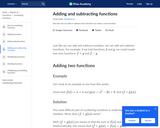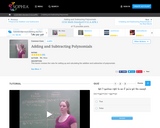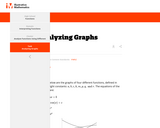Updating search results...

# 383 Results

View
Selected filters:
• math-1Conditional Remix & Share Permitted
CC BY-NC-SA
Rating
0.0 stars

F-IF.7: &nbsp;Analyze linear, exponential, and quadratic functions by generating different representations, by hand in simple cases and using technology for more complicated cases, to show key features, including: domain and range; rate of change; intercepts; intervals where the function is increasing, decreasing, positive, or negative; maximums and minimums; and end behavior.

Subject:
Mathematics
Material Type:
Activity/Lab
Author:
William Allred
Carrie Robledo
05/19/2021Rating
0.0 stars

In this article, students learn how to add or subtract two functions to create a new function.

Subject:
Mathematics
Material Type:
Demonstration
Provider:
02/28/2018Rating
0.0 stars

This lesson reviews the rules for setting up and calculating the addition and subtraction of polynomials.

Subject:
Mathematics
Material Type:
Demonstration
Provider:
Sophia
04/17/2018Rating
0.0 stars

This lesson will provide real world examples that involve adding and subtracting polynomials.

Subject:
Mathematics
Material Type:
Demonstration
Provider:
Sophia
04/17/2018Rating
0.0 stars

Students will learn how to combine like terms from two functions or take the difference of two functions.

Subject:
Mathematics
Material Type:
Demonstration
Provider:
Sophia
04/03/2018Rating
0.0 stars

This lesson covers the concept that when given two equations in the same format, those equations can be added or subtracted.

Subject:
Mathematics
Material Type:
Demonstration
Provider:
Sophia
04/03/2018Rating
0.0 stars

Lesson showing how to factor quadratics specifically when leading coefficient is not 1.

Subject:
Mathematics
Material Type:
Lesson Plan
Provider:
EngageNY
04/02/2018Rating
0.0 stars

Lesson with application of factoring quadratic expressions and word problems.

Subject:
Mathematics
Material Type:
Lesson Plan
Provider:
EngageNY
04/02/2018Rating
0.0 stars

In this lesson, students model cyclical phenomena from biological and physical science using trigonometric functions. Students understand that some periodic behavior is too complicated to be modeled by simple trigonometric functions.

Subject:
Mathematics
Material Type:
Lesson Plan
Provider:
EngageNY
08/15/2018Rating
0.0 stars

In this lesson, students summarize data on two categorical variables collected from a sample using a two-way frequency table. Given a two-way frequency table, students construct a relative frequency table and interpret relative frequencies.

Subject:
Mathematics
Material Type:
Lesson Plan
Provider:
EngageNY
08/15/2018Rating
0.0 stars

In this lesson, students distinguish between scatter plots that display a relationship that can be reasonably modeled by a linear equation and those that should be modeled by a nonlinear equation.

Subject:
Mathematics
Material Type:
Lesson Plan
Provider:
EngageNY
08/15/2018Rating
0.0 stars

In this lesson, students distinguish between scatter plots that display a relationship that can be reasonably modeled by a linear equation and those that should be modeled by a nonlinear equation. Students use an equation given as a model for a nonlinear relationship to answer questions based on an understanding of the specific equation and the context of the data.

Subject:
Mathematics
Material Type:
Lesson Plan
Provider:
EngageNY
08/15/2018Rating
0.0 stars

In this lesson, students determine the least-squares regression line from a given set of data using technology. Students use the least-squares regression line to make predictions.

Subject:
Mathematics
Material Type:
Lesson Plan
Provider:
EngageNY
08/13/2018Rating
0.0 stars

In this lesson, students use the least-squares line to predict values for a given data set. Students use residuals to evaluate the accuracy of predictions based on the least-squares line.

Subject:
Mathematics
Material Type:
Lesson Plan
Provider:
EngageNY
08/13/2018Rating
0.0 stars

In this lesson, students use the least-squares line to predict values for a given data set. Students use residuals to evaluate the accuracy of predictions based on the least-squares line.

Subject:
Mathematics
Material Type:
Lesson Plan
Provider:
EngageNY
08/13/2018Rating
0.0 stars

In this lesson, students use technology to determine the value of the correlation coefficient for a given data set. Students interpret the value of the correlation coefficient as a measure of strength and direction of a linear relationship. Students explain why correlation does not imply causation.

Subject:
Mathematics
Material Type:
Lesson Plan
Provider:
EngageNY
08/15/2018Rating
0.0 stars

In this lesson, students use data to develop a poster that involves the focus standards. Students construct a scatter plot of the data. Students analyze their data, examining the residual plot, and interpreting the correlation coefficient.

Subject:
Mathematics
Material Type:
Lesson Plan
Provider:
EngageNY
08/15/2018Rating
0.0 stars

Students will examine census data on marriage and divorce rates for women and men in each state and the District of Columbia. From these data, they will create a scatter plot, find a line of best fit, and analyze the relationship between the two variables (i.e., sex and marriage/divorce rates). They will also use a residual plot, explain the meaning of the slope and of the y-intercept of the line of best fit, and investigate the effect of outliers on this line.

Subject:
Mathematics
Material Type:
Activity/Lab
Provider:
U.S. Census Bureau
05/01/2018Conditional Remix & Share Permitted
CC BY-NC-SA
Rating
0.0 stars

The task could be used as a review problem or as an assessment problem after many different types of functions have been discussed. Since the different parameters of the functions are not given explicitly, the focus is not just on graphing specific functions but rather students have to focus on how values of parameters are reflected in a graph.

Subject:
Mathematics
Material Type:
Activity/Lab
Provider:
Illustrative Mathematics
03/28/2018